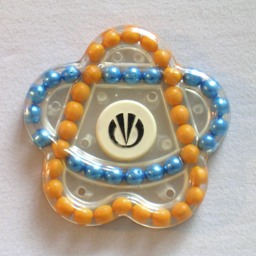# Starlet Puzzle / SwesdotschkaThis small Russian puzzle is usually called Swesdotschka, or Starlet, though it was originally produced under the name "Волшебные шарики" (Volshebnye shariki) which means "Magic beads". It consists of two intersecting rings made up of a number of coloured balls. The rings of balls intersect at four places, so they share four of the balls. Each ring of balls can be turned, so the balls can be mixed. The rings are shaped such that the puzzle as a whole looks like a five-pointed star.

One of the rings contains 26 balls, and is shaped a bit like the letter A. When solved, all its balls are yellow. The other ring has 23 balls, and will be blue when solved, except for the four balls it shares with the other ring. Let's call these rings A and B. The intersections divide each ring into four sections. Between the top two intersections A contains 5 balls, B contains 3 (excluding the balls at the intersections themselves), between the next two intersections A has 3, B has 5, between the next two A has 11, B has 6, and between the final pair A has 3, B has 5.

## The number of positions:

There are 45 balls, which can be arranged in at most 45! ways. This limit is not reached because:

• The yellow balls are indistinguishable (26!)
• The blue balls are indistinguishable (19!)

The total number of positions is therefore 45!/(26!·19!) = 2,438,362,177,020.

If your browser supports it, you can click on the link below to see a Javascript version of the Starlet puzzle:

## Notation:

Ring A is the ring shaped like an A, which is supposed to be yellow. The other ring is called ring B. Turning a ring a certain number of balls in the clockwise direction is denoted by the ring letter followed by the number of balls it is to move. A negative number is used to denote anti-clockwise turns of a ring.

## Solution:

1. If you can turn ring A to make 3 or 4 of the intersections blue, then do so.
2. If you can turn ring B to make 3 or 4 of the intersections yellow, then do so.
3. Repeat steps a-b as often as possible. This will quickly solve most of the balls.
4. Find a blue ball in ring A. Turn ring A to bring the blue ball to the top of the ring.
5. Find a yellow ball in the B ring (not at an intersection). If it lies on the left hand side, then turn over the puzzle so that it is on the right hand side.
6. Depending on where the yellow ball in ring B is located, do one of the following move sequences:
 Top section, middle ball: A3 B-2 A6 B2 A-6 B-2 Top section, right ball: A-3 B1 A-6 B-1 A6 B1 Right section, top ball: A-3 B-1 A-6 B1 A6 B-1 Right section, ball second from top: A-9 B-6 A-10 B6 Right section, middle ball: A1 B10 A-4 B-10 Right section, ball second from bottom: A3 B-4 A1 B4 A-1 B-4 Right section, bottom ball: A-7 B1 A12 B-1 A-12 B1 Bottom section, right ball: A13 B6 A-10 B-6 Bottom section, ball second from right: A-1 B4 A-6 B-6 A6 B2 Bottom section, ball third from right: A-11 B10 A4 B-10
7. Repeat d-f until ring A is completely yellow.